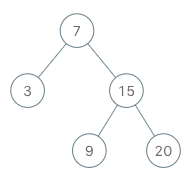# LeetCode 专题暴击：二叉树的iterative 做法汇总

### 知识点回顾

• 前序遍历：根节点 -> 左子树 -> 右子树
• 中序遍历：左子树 -> 根节点 -> 右子树
• 后序遍历：左子树 -> 右子树 -> 根节点

``````    1
/ \
2   3
/ \ / \
4  5 6  7``````
• 前序遍历：1 2 4 5 3 6 7
• 中序遍历：4 2 5 1 6 3 7
• 后序遍历：4 5 2 6 7 3 1

### 基础类型

↓点击题目就可以直接跳转到leetcode题目页面↓

#### 94. Binary Tree Inorder Traversal

test cases:``````Input: root = [1,null,2,3]
Output: [1,3,2]

Input: root = []
Output: []

Input: root = 
Output: ``````

``````
class Solution:
def inorderTraversal(self, root: TreeNode) -> List[int]:
res = []
def dfs(root):
if not root:
return
dfs(root.left)
res.append(root.val)
dfs(root.right)
dfs(root)
return res``````

• 先把root放入stack中
• 一直把root.left放入stack中，直到root.left为空
• 然后pop出stack中的元素，把元素的值放入res中
• 然后把root.right放入stack中，然后重复上面的操作
``````class Solution:
def inorderTraversal(self, root: TreeNode) -> List[int]:
res = []
stack = []
while root or stack: # 这里有两个条件，一个是root != None，一个是stack != []
while root: # 走到最左边
stack.append(root)
root = root.left
root = stack.pop()
res.append(root.val)
root = root.right
return res``````

• 时间复杂度：O(n)，因为每个节点都会被遍历一次
• 空间复杂度：O(n)，因为stack的大小最大为n

#### 144. Binary Tree Preorder Traversal

test cases:``````Input: root = [1,null,2,3]
Output: [1,2,3]

Input: root = []
Output: []

Input: root = 
Output: ``````

``````class Solution:
def preorderTraversal(self, root: TreeNode) -> List[int]:
res = []
def dfs(root):
if not root:
return
res.append(root.val)
dfs(root.left)
dfs(root.right)
dfs(root)
return res``````

• 先把root放入stack中
• 一直把root.left放入stack中，直到root.left为空
• 然后pop出stack中的元素
• 然后把root.right放入stack中，然后重复上面的操作
``````class Solution:
def preorderTraversal(self, root: TreeNode) -> List[int]:
res = []
stack = []
while root or stack:
while root:
res.append(root.val) # 因为前序遍历是先遍历根节点，所以这里先把root.val放入res中
stack.append(root)
root = root.left
root = stack.pop()
root = root.right
return res``````

• 时间复杂度：O(n)，因为每个节点都会被遍历一次
• 空间复杂度：O(n)，因为stack的大小最大为n

#### 145. Binary Tree Postorder Traversal

test cases:``````Input: root = [1,null,2,3]
Output: [3,2,1]

Input: root = []
Output: []

Input: root = 
Output: ``````

``````class Solution:
def postorderTraversal(self, root: TreeNode) -> List[int]:
res = []
def dfs(root):
if not root:
return
dfs(root.left)
dfs(root.right)
res.append(root.val)
dfs(root)
return res``````

• 先把root放入stack中
• 一直把root.left放入stack中，直到root.left为空
• 然后pop出stack中的元素
• 然后把root.right放入stack中，然后重复上面的操作
• 然后把root.val放入res中
``````class Solution:
def postorderTraversal(self, root: TreeNode) -> List[int]:
res = []
stack = []
while root or stack:
while root:
res.append(root.val) # 因为后序遍历是先遍历左子树，然后遍历右子树，然后遍历根节点，所以这里先把root.val放入res中
stack.append(root)
root = root.right
root = stack.pop()
root = root.left
return res[::-1]``````

• 时间复杂度：O(n)，因为每个节点都会被遍历一次
• 空间复杂度：O(n)，因为stack的大小最大为n

### 高级类型题

#### 173. Binary Search Tree Iterator

test cases:• 首先我们需要一个stack，然后我们把root放入stack中
• 然后我们一直把root.left放入stack中，直到root.left为空
• 然后我们pop出stack中的元素，然后把root.right放入stack中，然后重复上面的操作

``````class BSTIterator:
def __init__(self, root):
self.stack = []
while root:
self.stack.append(root)
root = root.left

# @return a boolean, whether we have a next smallest number
def hasNext(self):
return True if len(self.stack) else False

# @return an integer, the next smallest number
def next(self):
nxt = self.stack.pop()
x = nxt.right
while x:
self.stack.append(x)
x = x.left
return nxt.val``````

• 时间复杂度：O(n)，因为每个节点都会被遍历一次
• 空间复杂度：O(n)，因为stack的大小最大为n

#### 98. Validate Binary Search Tree

test cases:``````Input: root = [5,1,4,null,null,3,6]
Output: false
Explanation: The root node's value is 5 but its right child's value is 4.``````

• 首先我们需要一个stack，然后我们把root放入stack中
• 然后我们一直把root.left放入stack中，直到root.left为空
• 然后我们pop出stack中的元素，然后把root.right放入stack中，然后重复上面的操作
• 然后我们需要一个pre，来记录上一个节点的值，然后我们每次pop出stack中的元素的时候，我们都需要判断一下，当前的值是否大于pre，如果大于，那么我们就更新pre，然后继续往下走，如果不大于，那么我们就返回False
``````class Solution:
def isValidBST(self, root: TreeNode) -> bool:
stack = []
pre = float('-inf')
while root or stack:
while root:
stack.append(root)
root = root.left
root = stack.pop()
if root.val <= pre:
return False
pre = root.val
root = root.right
return True``````

• 时间复杂度：O(n)，因为每个节点都会被遍历一次
• 空间复杂度：O(n)，因为stack的大小最大为n